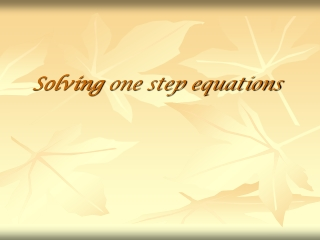DownloadDownload PresentationSolving one step equations

# Solving one step equations

Télécharger la présentation## Solving one step equations

- - - - - - - - - - - - - - - - - - - - - - - - - - - E N D - - - - - - - - - - - - - - - - - - - - - - - - - - -
##### Presentation Transcript

1. Solving one step equations

2. Properties of Equality • Addition: If a = b then a + c = b + c. 2. Subtraction: If a = b then a – c = b– c. 3. Multiplication: If a = b then ac = bc. 4. Division: If a = b and c ≠ 0 then a/c = b/c

3. Rules to Remember Quick Facts and Definitions: • When solving an equation, the goal is to get the variable by itself on one side of the equation. • Addition and subtraction are inverse operations. (opposites) • Multiplication and division are inverse operations. (opposites)

4. Solving Equations Requires BALANCE! Your equals sign will be the fulcrum (balancing point)! Just remember, whatever you do to one side must be done to the other side to maintain balance……..

5. Addition / Subtraction • If the number to move is positive, subtract it from both sides of the equation. • If the number to move is negative, add its positive value to both sides of the equation.

6. Solving with Addition Solve the following equation for x: x – 4 = 9 + 4+4 x = 13 We are able to get the x by itself by ADDING 4 to each side! (you WILL show this step in your homework!!)

7. Solving with Subtraction Solve the following equation for p: p + 7 = 21 - 7- 7 p = 14 We are able to get the p by itself by SUBTRACTING 7 from each side! (you WILL show this step in your homework!!)

8. Solving with Division Solve the following equation for m: 3 m = 18 • Divide both sides by 3 and simplify -- your work should look like this : • 3 m = 18 3 3 • m = 6 (you WILL show this step in your homework!!)

9. Solving with Multiplication Problems involving fractions are solved using multiplication, and the knowledge of multiplicative inverses (reciprocals)

10. Example : Solve the following equation for n: 2n = 3 5 7 • Multiply each side by the inverse of 25 : • 5•2n = 3•5 2 5 7 2 • n = 15 14

11. Your Turn: Solve the following equations with one step (show your work!!): • r + 4 = 17 • g - 15 = -11 • 13 + p = - 9 • -5b = 85 • - 7v = - 91 • w = -2 3 9

12. Answers: • r = 13 • g = 4 • p = - 22 • b = - 17 • v = 13 • w = - 2/3

13. Word Problems with One Step Equations You will need to know how to solve word problems involving these equations, by using the four-step problem solving plan: • Explore the problem • Plan the solution • Solve the problem • Examine the solution

14. 1. Explore the problem First, read the problem carefully, then: • Identify what information is given • Identify what you are asked to find

15. 2. Plan the Solution • Choose a variable to represent the unknown that you are trying to solve for • Create an algebraic equation for solving

16. Solve the Problem use algebraic methods Show your work! Examine the Solution Is your answer “reasonable” ? Did you check your work to be sure?

17. Let’s try it! Read the following: One mile is equal to approximately 1.6 kilometers. A local running club is holding a 10 kilometer race. What is the length of the race in miles? • Define aVariable Let m = the number of miles • 1.6 • m = 10 • Divide both sides by 1.6 (calculators ok) • m = 6.25 • The length of the race is 6.25 miles.

18. One more ! Write an Equation and Solve the Problem: Noah received a paycheck on Friday. After buying some athletic shoes for \$112, he had \$96 left. How much was his paycheck? • Let p = paycheck • p - 112 = 96 • + 112+112 • p = 208 • Noah’s paycheck was \$208.